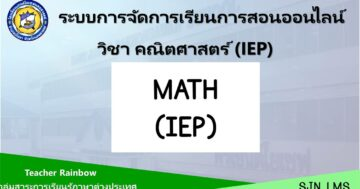# Mathematics IEP P.6 2nd Semester## เกี่ยวกับคอร์ส

Intensive Mathematics 6 Department of Mathematics
Primary 6 Time 40 Hours
Students are able to compare and arrange fractions and mixed numbers from different
situations; write the ratio to show the comparison of 2 amounts which are cardinal numbers from
given messages or situations; find the ratio which can be matched to the given ratio; find the
greatest common factor and the least common multiple of not more than 3 cardinal numbers;
show method of finding answers to problems by using the greatest common factor and the least
common multiple; find the answer for mix addition, subtraction, multiplication and division of
fractions and mixed numbers; show method of finding answers to 2-3 step problems of fractions
and mixed numbers; find the quotient of the decimal which the divisor and the quotient are the
decimals not more than 3 places; show the method of finding the answer to 3 steps addition,
subtraction, multiplication, and division decimal problems; show the method of thinking and finding
the answer to problems involving patterns; show the method of finding the answers to the
problems involving the volume of 3-D geometric shapes which are composed of cuboids; show
the method of finding the answer to the problems involving the perimeter and the area of polygon;
show the method of finding the answers to the problems involving the perimeter and the area of
a circle; classify a triangle by considering from its properties; construct a triangle when giving the
length of each side and the size of the angle; tell the characteristic of 3-D geometric shapes;
identify the 3-D geometric shapes which are from the unfolded shapes and identify the unfolded
shapes of 3-D geometric shapes; use the information from a pie chart to find the answer for the
problems.
These can be done through the determined situations for the students to learn, observe,
exercise their skills through the practices, summaries; to have good attitude towards mathematics;
to be creative; to develop their skills and mathematical interpretation; and be able to use in their
daily life.

Indicators
M1.1 Gr6/1 Gr6/2 Gr6/3 Gr6/4 Gr6/5
Gr6/6 Gr6/7 Gr6/8 Gr6/9 Gr6/10
Gr6/11 Gr6/12
M1.2 Gr6/1
M2.1 Gr6/1 Gr6/2 Gr6/3
M2.2 Gr6/1 Gr6/2 Gr6/3 Gr6/4
M3.1 Gr6/1
Total 21 indicators

### What Will You Learn?

• 1. Students are able to find the answers to the problems involving the perimeter and the area of
• a circle; classify a triangle by considering from its properties
• 2. Students are able to construct a triangle when giving the
• length of each side and the size of the angle; tell the characteristic of 3-D geometric shapes.
• 3. Students are able to use the information from a pie chart to find the answer for the
• problems.

00:00
00:00

00:00
00:00
00:00

### Student Ratings & ReviewsNo Review Yet